Courses

# Test: Shear Force & Bending Moment - 1

## 10 Questions MCQ Test Topicwise Question Bank for Civil Engineering | Test: Shear Force & Bending Moment - 1

Description
This mock test of Test: Shear Force & Bending Moment - 1 for Civil Engineering (CE) helps you for every Civil Engineering (CE) entrance exam. This contains 10 Multiple Choice Questions for Civil Engineering (CE) Test: Shear Force & Bending Moment - 1 (mcq) to study with solutions a complete question bank. The solved questions answers in this Test: Shear Force & Bending Moment - 1 quiz give you a good mix of easy questions and tough questions. Civil Engineering (CE) students definitely take this Test: Shear Force & Bending Moment - 1 exercise for a better result in the exam. You can find other Test: Shear Force & Bending Moment - 1 extra questions, long questions & short questions for Civil Engineering (CE) on EduRev as well by searching above.
QUESTION: 1

### For the loaded beam shown in the figure, the correct shear force diagram is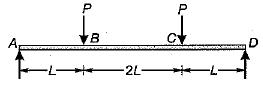Solution:

The shear force in the span BC will be zero. The shear force in the span AB and CD will be of opposite sign.

QUESTION: 2

### For the simply supported beam, shown in the figure below at what distance from the support A is the shear force zero?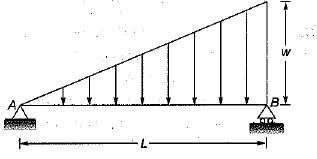Solution: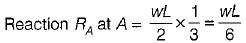Let the point of zero shear force occur at section X-Xfrom left support A
∴ Shear force at X-X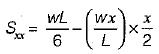∴ For zero shear force we have,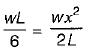∴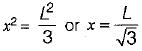QUESTION: 3

### For the beam shown ih the given figure, the maximum positive bending moment is equal to the maximum negative bending moment. The value of L1 is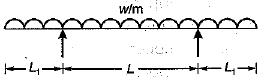Solution:

The BM can be found from the area of SFD.
The shear force diagram is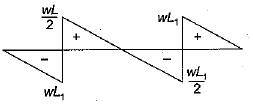Maximum negative, bending moment,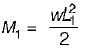Maximum positive, bending moment,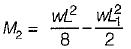For M1 = M2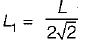QUESTION: 4

The maximum bending moment due to a moving load on a fixed ended beam occurs .

Solution:
QUESTION: 5

If a beam is subjected to a constant bending moment along its length then the shear force will

Solution:

If M is constant then,
S.F = 0, as
S.F = dM/dx

QUESTION: 6

If the shear force acting at every section of a beam is of the same magnitude and of the same direction, then it represents a

Solution: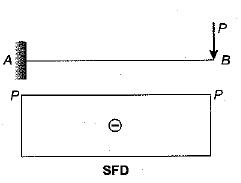QUESTION: 7

The shear force diagram shown in the figure is that of a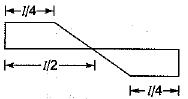Solution:
QUESTION: 8

If the bending moment diagram for a simply suppoted beam is of the form as given in figure then the load acting on the beam is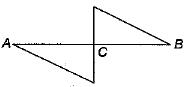Solution: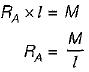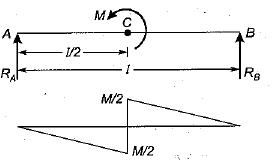Span AC: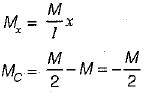Span CB: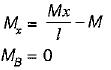QUESTION: 9

A beam is simply supported at its ends and is loaded by a couple at its mid-span as shown in figure. Shear force diagram for the beam is given by the figure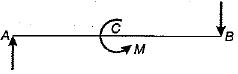Solution: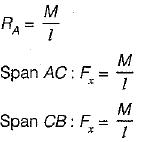QUESTION: 10

A horizontal beam carrying uniformly distributed load is supported with equal overhangs as shown in the figure beiow. The resultant bending moment at the mid-span shall be zero if a/b is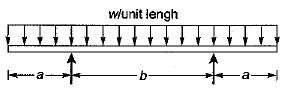Solution:

Since both the support reactions and loadings are symmetrically place about the center both the support will be of same magnitude and equal to ½ of total load acting on beam.
RA = RB = [w (2a+b)] / 2
= (w (2a+b))/2=w(a+b/2)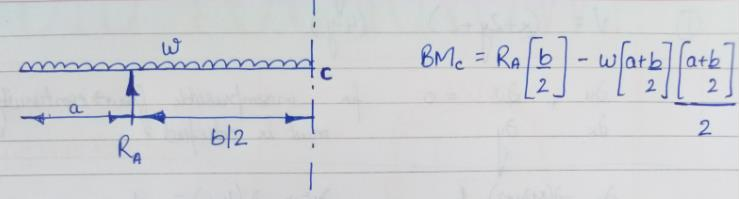From the fig. bending moment at center (C) i.e. mid-span will be equal to:
(RA) (b/2) – w (a+b/2)( ((a+b/2))/2 ) = BMc =0
w(a+b/2) (b/2) – w (a+b/2)( ((a+b/2))/2 ) =0
b−(a+b/2)=0
b = 2a
a/b  = 1/2